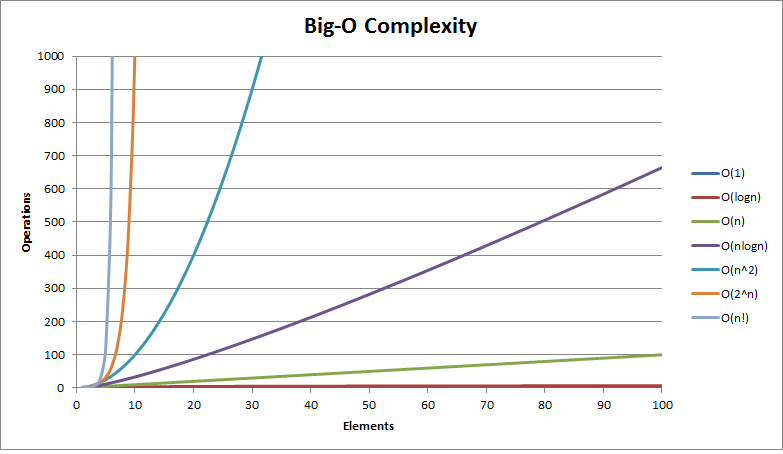f(n) 释义
O(1) 常数阶
O(n) 线性阶
O(log(n)) 对数阶
O(n²) 平方阶

# 图例

 绝佳 不错 一般 不佳 糟糕

# 数据结构操作

平均最差最差
访问搜索插入删除访问搜索插入删除
Array-【数组】 O(1) O(n) O(n) O(n) O(1) O(n) O(n) O(n) O(n)
Stack-【栈】 O(n) O(n) O(1) O(1) O(n) O(n) O(1) O(1) O(n)
Singly-Linked List-【单链表】 O(n) O(n) O(1) O(1) O(n) O(n) O(1) O(1) O(n)
Doubly-Linked List-【双向链表】 O(n) O(n) O(1) O(1) O(n) O(n) O(1) O(1) O(n)
Skip List-【跳表】 O(log(n)) O(log(n)) O(log(n)) O(log(n)) O(n) O(n) O(n) O(n) O(n log(n))
Hash Table-【Hash表】 - O(1) O(1) O(1) - O(n) O(n) O(n) O(n)
Binary Search Tree-【二叉查找树】 O(log(n)) O(log(n)) O(log(n)) O(log(n)) O(n) O(n) O(n) O(n) O(n)
Cartesian Tree-【笛卡尔积树】 - O(log(n)) O(log(n)) O(log(n)) - O(n) O(n) O(n) O(n)
B-Tree-【多路搜索树】 O(log(n)) O(log(n)) O(log(n)) O(log(n)) O(log(n)) O(log(n)) O(log(n)) O(log(n)) O(n)
Red-Black Tree-【红黑树】 O(log(n)) O(log(n)) O(log(n)) O(log(n)) O(log(n)) O(log(n)) O(log(n)) O(log(n)) O(n)
Splay Tree-【伸展树】 - O(log(n)) O(log(n)) O(log(n)) - O(log(n)) O(log(n)) O(log(n)) O(n)
AVL Tree-【自平衡二叉查找树】 O(log(n)) O(log(n)) O(log(n)) O(log(n)) O(log(n)) O(log(n)) O(log(n)) O(log(n)) O(n)

# 数组排序算法

最佳平均最差最差
Quicksort-【快速排序】 O(n log(n)) O(n log(n)) O(n^2) O(log(n))
Mergesort-【归并排序】 O(n log(n)) O(n log(n)) O(n log(n)) O(n)
Timsort-【优化版归并排序】 O(n) O(n log(n)) O(n log(n)) O(n)
Heapsort-【堆排序】 O(n log(n)) O(n log(n)) O(n log(n)) O(1)
Bubble Sort-【冒泡排序】 O(n) O(n^2) O(n^2) O(1)
Insertion Sort-【插入排序】 O(n) O(n^2) O(n^2) O(1)
Selection Sort-【选择排序】 O(n^2) O(n^2) O(n^2) O(1)
Shell Sort-【希尔排序】 O(n) O((nlog(n))^2) O((nlog(n))^2) O(1)
Bucket Sort-【桶排序】 O(n+k) O(n+k) O(n^2) O(n)
Radix Sort-【基数排序】 O(nk) O(nk) O(nk) O(n+k)

# 图操作

Adjacency list-【邻接表】 O(|V|+|E|) O(1) O(1) O(|V| + |E|) O(|E|) O(|V|)
Incidence list-【关联表】 O(|V|+|E|) O(1) O(1) O(|E|) O(|E|) O(|E|)
Adjacency matrix-【邻接矩阵】 O(|V|^2) O(|V|^2) O(1) O(|V|^2) O(1) O(1)
Incidence matrix-【关联矩阵】 O(|V| ⋅ |E|) O(|V| ⋅ |E|) O(|V| ⋅ |E|) O(|V| ⋅ |E|) O(|V| ⋅ |E|) O(|E|)

# 堆操作

Heapify查找最大值分离最大值提升键插入删除合并
Linked List (sorted) - O(1) O(1) O(n) O(n) O(1) O(m+n)
Linked List (unsorted) - O(n) O(n) O(1) O(1) O(1) O(1)
Binary Heap-【二叉堆】 O(n) O(1) O(log(n)) O(log(n)) O(log(n)) O(log(n)) O(m+n)
Binomial Heap-【二项堆】 - O(1) O(log(n)) O(log(n)) O(1) O(log(n)) O(log(n))
Fibonacci Heap-【斐波那契堆】 - O(1) O(log(n)) O(1) O(1) O(log(n)) O(1)

# 大 O 复杂度图表# `总结` 四张常见的时间复杂度的用时如下：

O(1)< O(log(n))< O(n)< O(n^2)

# 另:编程的世界中还有各种各样的算法，除了上述四种，还有许多不用形式的时间复杂度:

O(n(log(n))),O(n^3),O(m*n),O(2^n),O(n!)